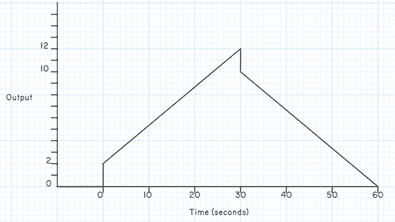# Proportional plus derivative controller

• Engineering
Jason-Li
Homework Statement:
FIGURE 6 shows a proportional plus derivative controller that has a proportional band of 20% and a derivative action time of 0.1 minutes. Construct the shape of the output waveform for the triangular input waveform shown, if the input rises and falls at the rate of 4 units per minute.
Relevant Equations:
None required
Hello,

There is a thread related to this question however it was marked correct but doesn't look correct to me?

I think I have it but it is quite different to other answers I have seen?
I have found
PB=20% hence gain=5
dAT = 0.1min = 6s
Would rise and peak at 2units at 30s however due to gain this will be 10units, hence change due to derivative would be:
10/30 * 6s = 2units, then once peaked at 30s it would reduce by the same derivative and create the following graph:Mentor
Find out information about proportional-plus-derivative control. Control in which the control signal is a linear combination of the error signal and its derivative.
Can you post some links to such information that would be helpful for the Original Poster (OP)? Thanks.

Jason-Li
@spoto2 @berkeman

Sorry I've looked at a few different sources but my graph still looks correct to me, any advice?

Mentor
Not one of my areas of expertise, unfortunately. Jim is no longer with us (RIP), but @rude man still is at PF...Tromso80
Hi Jason, Just wondered what your maths is behind this?

Jason-Li
Hi Jason, Just wondered what your maths is behind this?

The maths is in the first post, which part are you unsure of?